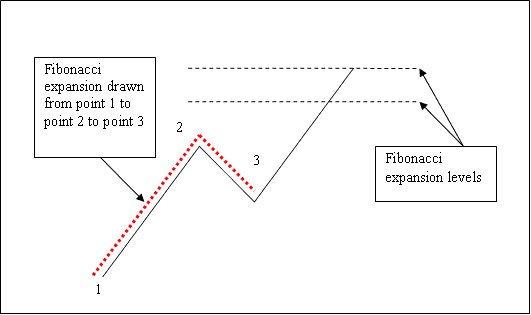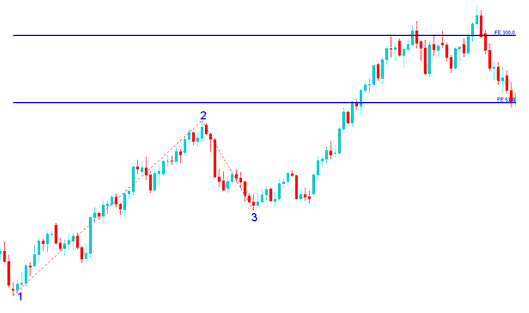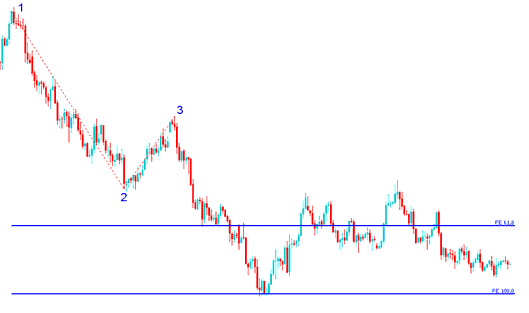# How to Draw Indices Trading Fibonacci Projection Indicator Tool on Stock Indexes Charts

## How Do I Use Indices Trading Fibonacci Projection Levels on Indices Chart?

We use Fibonacci Projectionlevels to estimate where the indices trend movement will reach. There are 2 important Fibonacci Projection levels; 61.8% and 100% Fibonacci Projection Levels, these are used for taking profit.

To draw Fibonacci Projection levels on the stock indices chart we wait until the stock indexes price retracement is complete and the stock indexes price starts to move in the original direction of the Indices trend. Where the stock indexes price retracement reaches is used as chart point 3. Fibonacci Projection Indicator is drawn using three chart points as shown on the stock indexes trading example explained and illustrated below of how to draw the Fibonacci Projection Indicator Tool.

The Fibonacci Projection example explained and illustrated below shows the 3 Chart Points where the Fibonacci Projection indicator is drawn, marked as Chart point 1, Chart point 2 and Chart point 3.

Chart point 1 is where the indices trend started, Chart point 2 is where the indices trend pulled back and retraced and Chart point 3 is where the stock indices retracement reached as shown on the Fibonacci Projection Indicator Tool example explained and illustrated below.How To Trade With Fibonacci Projection? - How to Draw Fibonacci Projection Levels on Indices Charts

Please note where these Fibonacci Projection levels are drawn - Fibonacci Projection levels are drawn above the Fibonacci indicator, these are the chart points where a indices trader will set the take profit orders using these Fibonacci Projection Levels - 61.8% and 100.0% Fibonacci Projection Levels.

## Drawing Fibonacci Projection Levels on an Upward Indices Trend

We use Fibonacci Projectionlevels to estimate where the indices trend movement will reach. There are 2 important Fibonacci Projection levels; 61.8%Fibonacci Projection Level and 100% Fibonacci Projection Level, these Fibonacci Projection Levels are used for taking profit.

On the Fibonacci Projection indicator example explained and illustrated below you can see that the Fibonacci Projection indicator tool is drawn along the direction of the indices trend, since the indices trend is upwards - the Fibonacci Projection indicator is drawn upwards.

These Fibonacci Projection levels are displayed as horizontal lines above the Fibonacci Projection indicator, showing profit taking areas. In the stock indexes trading example explained and illustrated below if you had used of 100.0% Fibonacci Projection level you would have made nice profit from the trade setup.Drawing Fibonacci Projection Indicator on an Upward Indices Trend - Fibonacci Projection Trading Tool

From the above Fibonacci Projection indicator example, the upward indices trend continued and both 61.8% and 100.0% Fibonacci Projection levels were all hit after which stock indexes price retraced again after getting to the 100.0 % Fibonacci Projection level.

## Drawing Fibonacci Projection Levels on a Downward Indices Trend

Since we use the Fibonacci Projection indicator tool to estimate take profit levels, how do we draw Fibonacci Projection in a downward Indices trend?

We draw the Fibonacci Projection indicator tool from chart point 1 to chart point 2 to chart point 3 as shown on the stock indexes trading example explained and illustrated below. Remember we always draw this Fibonacci Projection tool in the direction of the indices trend. In the Fibonacci Projection example explained and illustrated below, can you figure what direction we have drawn it? That is right - downward direction.

Try and spot the difference between how we have drawn Fibonacci Projection indicator above and how Fibonacci Projection indicator is drawn below. This time you would also have used Fibonacci Projection level 100.0%, just where the stock indexes price reached as shown on the stock indexes trading example explained and illustrated below. That would have been a good take profit area.Drawing Fibonacci Projection Indicator on a Downward Indices Trend - Fibonacci Projection Trading Tool

From the Fibonacci Projection indicator example above, after drawing this Fibonacci Projection tool there are two levels that are used to show the profit taking areas, these two Fibonacci Projection levels are drawn as horizontal lines across the stock indexes price chart marked as 61.8%Fibonacci Projection Level and 100% Fibonacci Projection Level.